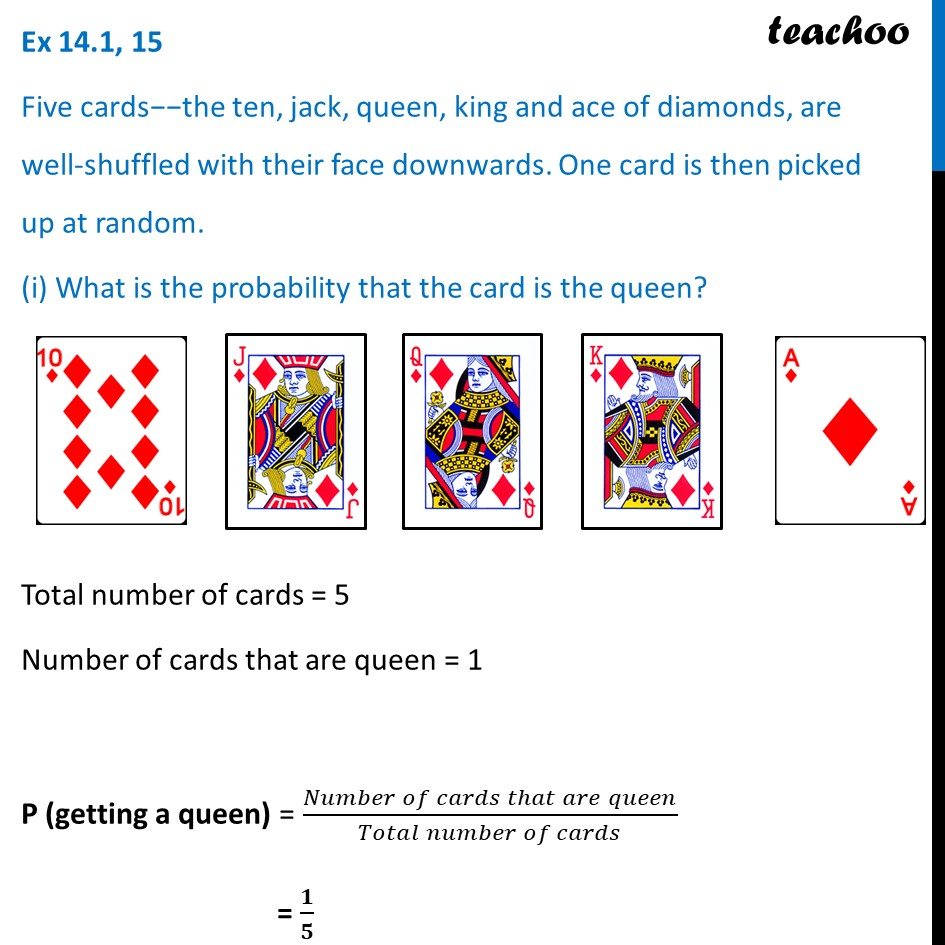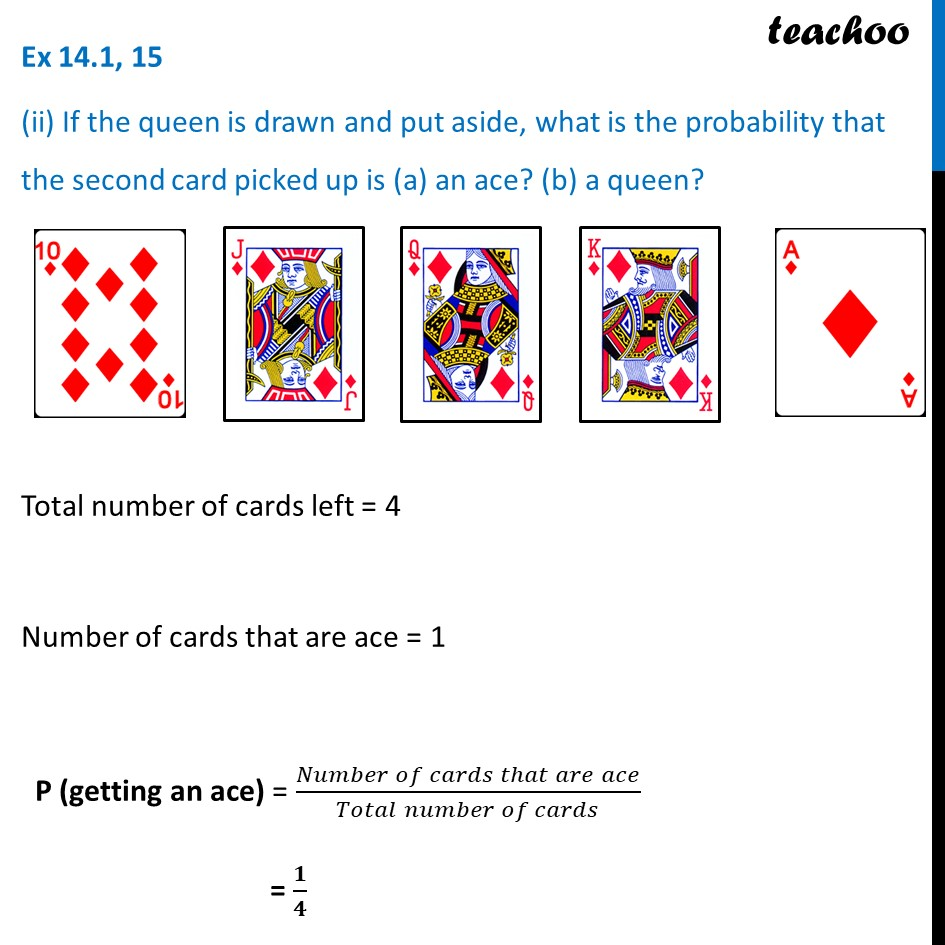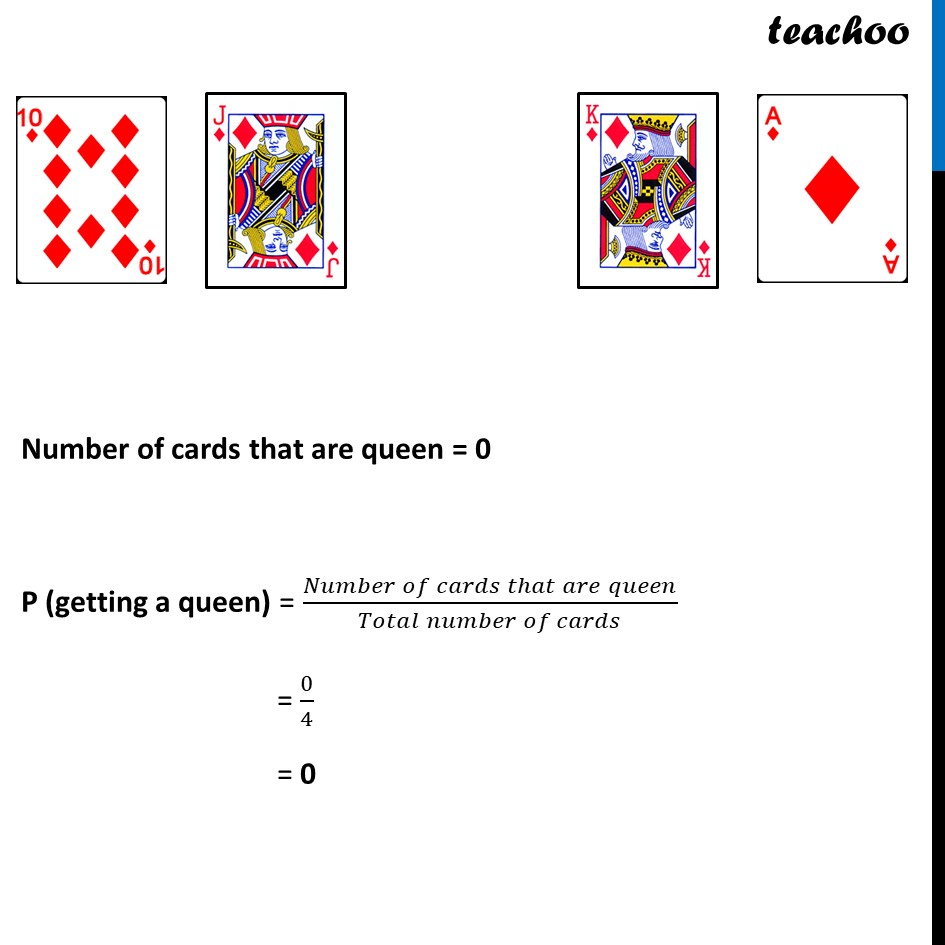Chapter 15 Class 10 Probability

Class 10
Important Questions for Exam - Class 10Learn in your speed, with individual attention - Teachoo Maths 1-on-1 Class

### Transcript

Ex 14.1, 15 Five cards−−the ten, jack, queen, king and ace of diamonds, are well-shuffled with their face downwards. One card is then picked up at random. (i) What is the probability that the card is the queen? Total number of cards = 5 Number of cards that are queen = 1 P (getting a queen) = (𝑁𝑢𝑚𝑏𝑒𝑟 𝑜𝑓 𝑐𝑎𝑟𝑑𝑠 𝑡ℎ𝑎𝑡 𝑎𝑟𝑒 𝑞𝑢𝑒𝑒𝑛)/(𝑇𝑜𝑡𝑎𝑙 𝑛𝑢𝑚𝑏𝑒𝑟 𝑜𝑓 𝑐𝑎𝑟𝑑𝑠) = 𝟏/𝟓 Ex 14.1, 15 (ii) If the queen is drawn and put aside, what is the probability that the second card picked up is (a) an ace? (b) a queen? Total number of cards left = 4 Number of cards that are ace = 1 P (getting an ace) = (𝑁𝑢𝑚𝑏𝑒𝑟 𝑜𝑓 𝑐𝑎𝑟𝑑𝑠 𝑡ℎ𝑎𝑡 𝑎𝑟𝑒 𝑎𝑐𝑒)/(𝑇𝑜𝑡𝑎𝑙 𝑛𝑢𝑚𝑏𝑒𝑟 𝑜𝑓 𝑐𝑎𝑟𝑑𝑠) = 𝟏/𝟒 Number of cards that are queen = 0 P (getting a queen) = (𝑁𝑢𝑚𝑏𝑒𝑟 𝑜𝑓 𝑐𝑎𝑟𝑑𝑠 𝑡ℎ𝑎𝑡 𝑎𝑟𝑒 𝑞𝑢𝑒𝑒𝑛)/(𝑇𝑜𝑡𝑎𝑙 𝑛𝑢𝑚𝑏𝑒𝑟 𝑜𝑓 𝑐𝑎𝑟𝑑𝑠) = 0/4 = 0